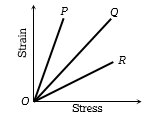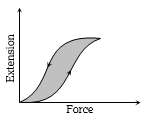If the Young's modulus of the material is 3 times its modulus of rigidity, then its volume elasticity will be

(a) Zero                              (b) Infinity

(c) $2×{10}^{10}N/{m}^{2}$           (d) $3×{10}^{10}N/{m}^{2}$

High Yielding Test Series + Question Bank - NEET 2020

Difficulty Level:

Modulus of rigidity of a liquid

(a) Non zero constant

(b) Infinite

(c) Zero

(d) Can not be predicted

Difficulty Level:

A cube of aluminium of sides 0.1 m is subjected to a shearing force of 100 N. The top face of the cube is displaced through 0.02 cm with respect to the bottom face. The shearing strain would be

(a) 0.02                                   (b) 0.1

(c) 0.005                                 (d) 0.002

High Yielding Test Series + Question Bank - NEET 2020

Difficulty Level:

The upper end of a wire of radius 4 mm and length 100 cm is clamped and its other end is twisted through an angle of 30°. Then angle of shear is

(a) $12°$                                      (b) $0.12°$

(c) $1.2°$                                     (d) $0.012°$

Concept Questions :-

Stress-strain
High Yielding Test Series + Question Bank - NEET 2020

Difficulty Level:

A rod of length l and radius r is joined to a rod of length l/2 and radius r/2 of same material. The free end of small rod is fixed to a rigid base and the free end of larger rod is given a twist of $\theta$, the twist angle at the joint will be

(a) $\theta /4$                          (b) $\theta /2$

(c) $5\theta /6$                         (d) $8\theta /9$

Concept Questions :-

Stress-strain
High Yielding Test Series + Question Bank - NEET 2020

Difficulty Level:

Shearing stress causes a change in-

(c)   Shape                                (d)   Volume

Concept Questions :-

Stress-strain

Difficulty Level:

To break a wire, a force of ${10}^{6}N/{m}^{2}$ is required. If the density of the material is , then the length of the wire which will break by its own weight will be -

(a) 34 m                             (b) 30 m

(c) 300 m                            (d) 3 m

Concept Questions :-

Stress-strain
High Yielding Test Series + Question Bank - NEET 2020

Difficulty Level:

One end of a uniform wire of length L and of weight $W$ is attached rigidly to a point in the roof and a weight ${W}_{1}$ is suspended from its lower end. If S is the area of cross-section of the wire, the stress in the wire at a height 3L/4 from its lower end is

1. $\frac{{W}_{1}}{S}$                                  2. $\frac{{W}_{1}+\left(W/4\right)}{S}$

3. $\frac{{W}_{1}+\left(3W/4\right)}{S}$                    4. $\frac{{W}_{1}+W}{S}$

Concept Questions :-

Stress-strain
High Yielding Test Series + Question Bank - NEET 2020

Difficulty Level:

The strain-stress curves of three wires of different materials are shown in the figure. P, Q and R are the elastic limits of the wires. The figure shows that(a) Elasticity of wire P is maximum

(b) Elasticity of wire Q is maximum

(c) Tensile strength of R is maximum

(d) None of the above is true

Concept Questions :-

Stress-strain curve
High Yielding Test Series + Question Bank - NEET 2020

Difficulty Level:

The diagram shows a force-extension graph for a rubber band. Consider the following statementsI. It will be easier to compress this rubber than expand it

II. Rubber does not return to its original length after it is stretched

III. The rubber band will get heated if it is stretched and released

Which of these can be deduced from the graph?

(a)   III only                               (b)   II and III

(c)   I and III                             (d)   I only

Concept Questions :-

Stress-strain curve